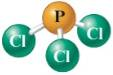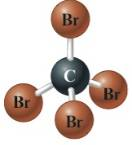Chapter 4, Problem 22ALQIntroductory Chemistry: A Foundati...

9th Edition
Steven S. Zumdahl + 1 other
ISBN: 9781337399425

Solutions

Chapter
SectionIntroductory Chemistry: A Foundati...

9th Edition
Steven S. Zumdahl + 1 other
ISBN: 9781337399425
Textbook Problem

Write the formula for each of the following substances, listing the elements in the order given.List the phosphorus atom first.a molecule containing two boron atoms and six hydrogen atomsa compound containing one calcium atom for every two chlorine atomsList the carbon atom first.a compound containing two iron atoms for every three oxygen atomsa molecule containing three hydrogen atoms, one phosphorus atom, and four oxygen atoms

Interpretation Introduction

(a)

Interpretation:

The chemical formula of the compound should be determined.

Concept Introduction:

There are following general rules for writing the formula of compound from their structures.

1. The element symbol is used to represent an element. It is first or first two from the name of the elements in the periodic table.
2. The number of atoms of the elements can be represented as subscript in the element symbol.
3. When the number of atoms is only one then subscript (1) is not written.
Explanation

The symbols of atoms are given. From the structure, the number of phosphorus P atoms ...

Interpretation Introduction

(b)

Interpretation:

The chemical formula of the compound should be determined.

Concept Introduction:

There are following general rules for writing the formula of compound from their structures.

1. The element symbol is used to represent an element. It is first or first two from the name of the elements in the periodic table.
2. The number of atoms of the elements can be represented as subscript in the element symbol.
3. When the number of atoms is only one then subscript (1) is not written.
Interpretation Introduction

(c)

Interpretation:

The chemical formula of the compound should be determined.

Concept Introduction:

There are following general rules for writing the formula of compound from their structures.

1. The element symbol is used to represent an element. It is first or first two from the name of the elements in the periodic table.
2. The number of atoms of the elements can be represented as subscript in the element symbol.
3. When the number of atoms is only one then subscript (1) is not written.
Interpretation Introduction

(d)

Interpretation:

The chemical formula of the compound should be determined.

Concept Introduction:

There are following general rules for writing the formula of compound from their structures.

1. The element symbol is used to represent an element. It is first or first two from the name of the elements in the periodic table.
2. The number of atoms of the elements can be represented as subscript in the element symbol.
3. When the number of atoms is only one then subscript (1) is not written.
Interpretation Introduction

(e)

Interpretation:

The chemical formula of the compound should be determined.

Concept Introduction:

There are following general rules for writing the formula of compound from their structures.

1. The element symbol is used to represent an element. It is first or first two from the name of the elements in the periodic table.
2. The number of atoms of the elements can be represented as subscript in the element symbol.
3. When the number of atoms is only one then subscript (1) is not written.
Interpretation Introduction

(f)

Interpretation:

The chemical formula of the compound should be determined.

Concept Introduction:

There are following general rules for writing the formula of compound from their structures.

1. The element symbol is used to represent an element. It is first or first two from the name of the elements in the periodic table.
2. The number of atoms of the elements can be represented as subscript in the element symbol.
3. When the number of atoms is only one then subscript (1) is not written.

Still sussing out bartleby?

Check out a sample textbook solution.

See a sample solution

The Solution to Your Study Problems

Bartleby provides explanations to thousands of textbook problems written by our experts, many with advanced degrees!

Get Started